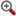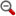# 一文教你使用HSV颜色模型和openCV构建昼夜分类器发布日期：2021-08-11 12:00:25   浏览次数：38

﻿

Pythonpipopencvnumpymatplotlib

Opencv 是一个计算机视觉包,我们将使用它来处理图像和操作它。numpy用于数值计算,matplotlib用于图像显示。

1． 导入用于测试的库和图像

``from util import daynight_helperimport matplotlib．pyplot as pltimport cv2import numpy as np #path to the folder where the images aretraining_data_path = 'data/day_night_images/training'``
2． 预处理图像及其标签

``data /   day_night_images /    training /      day /        img001．jpg        img002．jpg      night /         img100．jpg        img101．jpg    test /      day /        img001．jpg        img002．jpg      night /         img100．jpg        img101．jpg``

``import osimport glob import matplotlib．image as mpimgimport cv2def load_dataset(image_dir):    img_list = []    img_types = ['day', 'night']        for img_type in img_types:                for file in glob．glob(os．path．join(image_dir, img_type, '*')):            img = mpimg．imread(file)                        if not img is None:                img_list．append((img, img_type))                        return img_listdef standardize_image(image):    std_img=  cv2．resize(image, (1110, 600))       return std_imgdef encode_label(label):    if label== 'day':        return 1    else:        return 0def standardize_inputs(img_list):    std_list = []        for img in img_list:        std_img = standardize_image(img)        std_label = encode_label(img)                std_list．append((std_img, std_label))        return std_list       ``

``training_data_path = 'data/day_night_images/training'training_data = daynight_helper．load_dataset(training_data_path)std_training_data = daynight_helper．standardize_inputs(training_data)img = std_training_datalabel = std_training_dataplt．imshow(img)print('Shape ', img．shape)print('label: ', label)``

3．获取图片的平均亮度

``#feature extraction - brightness using hsvdef brightness_value(img):    hsv_img = cv2．cvtColor(img, cv2．COLOR_RGB2HSV)    v_values = np．sum(hsv_img[:, :, 2])    area = img．shape * img．shape    avg_brightness = v_values/area    return avg_brightness``

4． 使用选定的标记昼夜差异的阈值来估计标签

``def estimate_label(img, threshold):    avg_brightness = brightness_value(img)    predicted_label = 0    threshold = threshold    if avg_brightness > threshold:        predicted_label = 1        return predicted_label, avg_brightness``

5． 寻找最优阈值

``def estimate_label(img, threshold):    avg_brightness = brightness_value(img)    predicted_label = 0    threshold = threshold    if avg_brightness > threshold:        predicted_label = 1        return predicted_label, avg_brightness``

``threshold = 120for i in range(0, len(std_training_data)):    img_data = std_training_data[i]    threshold = optimize_threshold(img_data, threshold)print('threshold ', threshold)#threshold  116``

PS:可以以不同的方式调整阈值,即平均阈值和平均亮度。这是我在这段代码中使用的技术。

6． 在测试图像上运行分类器

``import randomtest_data_path = 'data/day_night_images/test'test_data = daynight_helper．load_dataset(test_data_path)test_data = daynight_helper．standardize_inputs(test_data)random．shuffle(test_data)threshold = 116correctly_classified = []misclassified = []for i in range(0, len(test_data)):    img_data = test_data[i]    pred, avg_brightness = estimate_label(img_data, threshold)    #print('predicted ', pred)    label = img_data    #print('label ', label)    if pred == label:        correctly_classified．append(img_data)    else:         misclassified．append(img_data)        #total :  160#Correct predictions:  140#Misclassified:  20#Accuracy  0．875``

[ 智能网搜索 ]  [ 打印本文 ]  [ 违规举报

﻿

﻿